Anzeige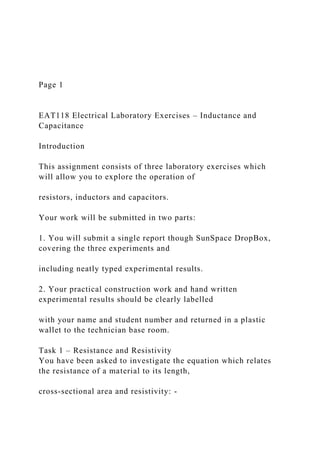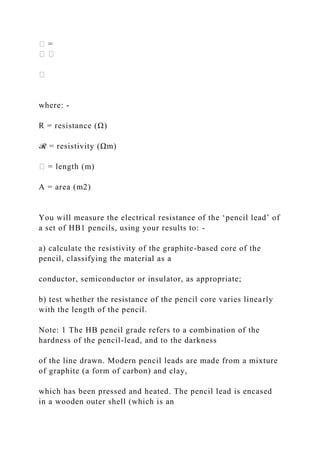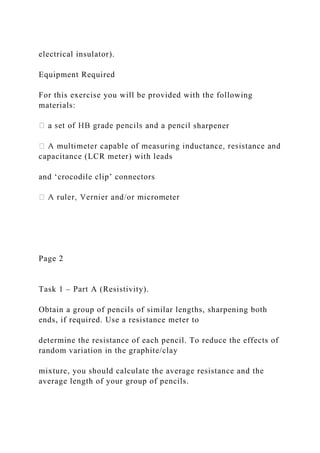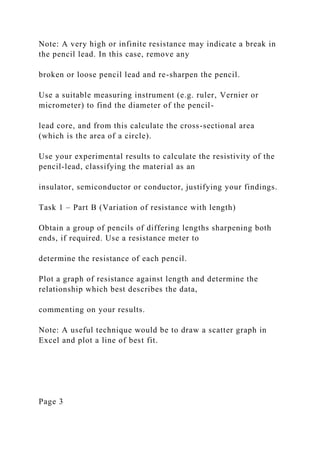Anzeige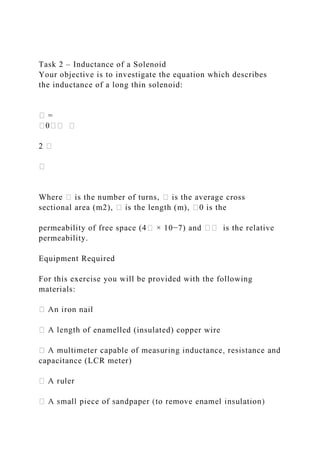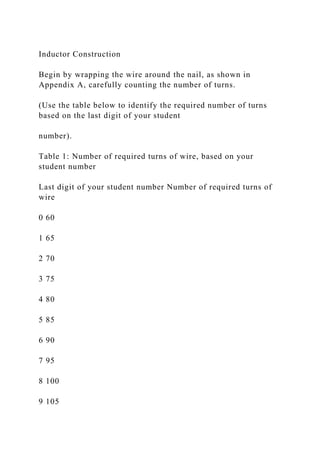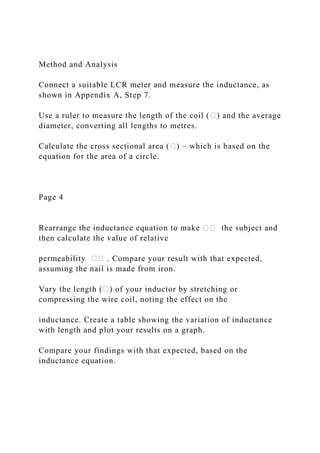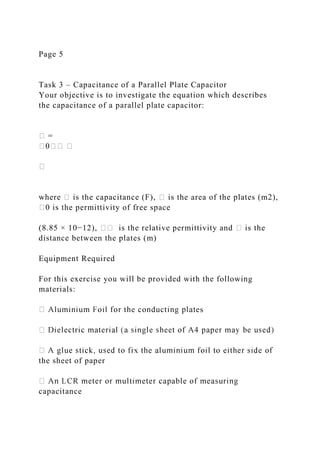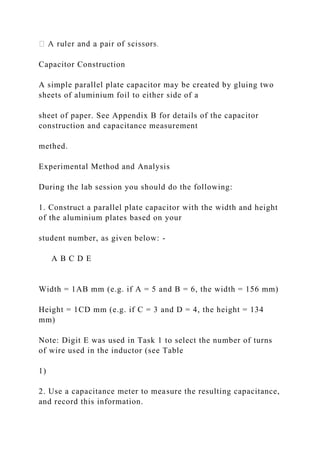Anzeige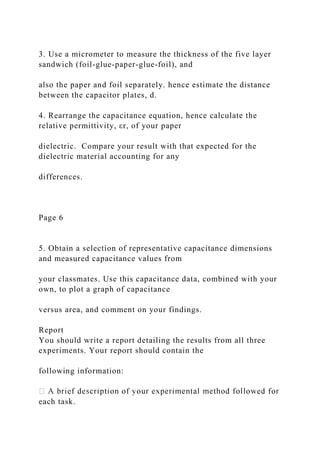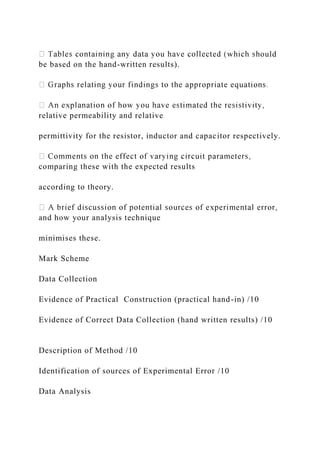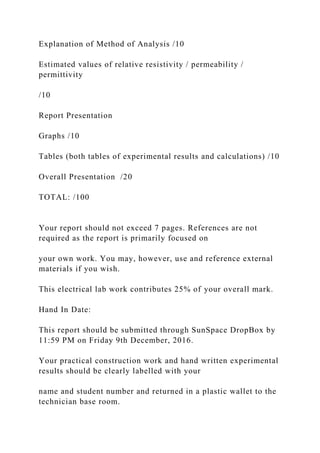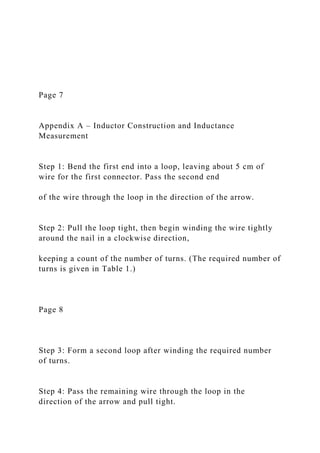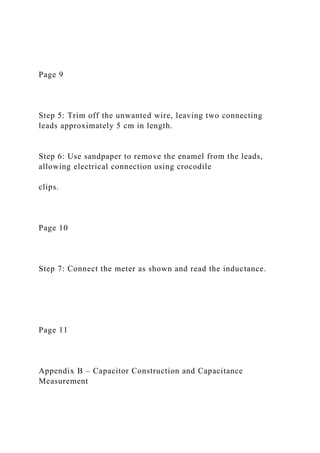Anzeige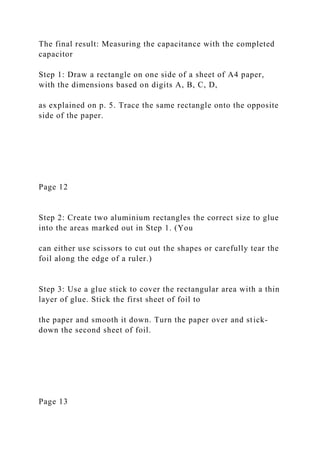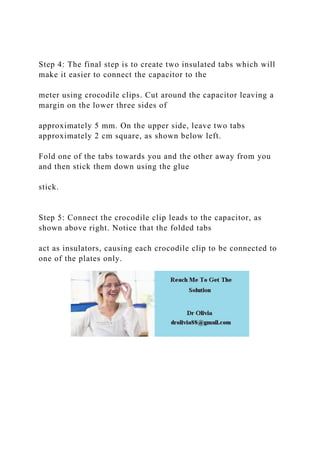Nächste SlideShare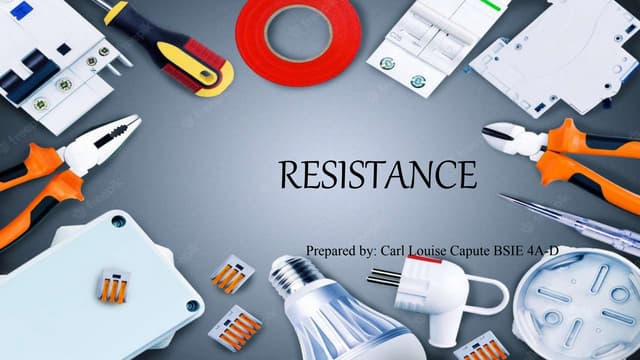RESISTANCE.pptx
1 von 16
Anzeige

### Page 1 EAT118 Electrical Laboratory Exercises – Inducta.docx

1. Page 1 EAT118 Electrical Laboratory Exercises – Inductance and Capacitance Introduction This assignment consists of three laboratory exercises which will allow you to explore the operation of resistors, inductors and capacitors. Your work will be submitted in two parts: 1. You will submit a single report though SunSpace DropBox, covering the three experiments and including neatly typed experimental results. 2. Your practical construction work and hand written experimental results should be clearly labelled with your name and student number and returned in a plastic wallet to the technician base room. Task 1 – Resistance and Resistivity You have been asked to investigate the equation which relates the resistance of a material to its length, cross-sectional area and resistivity: -
2. � = � � � where: - R = resistance (Ω) ℛ = resistivity (Ωm) � = length (m) A = area (m2) You will measure the electrical resistance of the ‘pencil lead’ of a set of HB1 pencils, using your results to: - a) calculate the resistivity of the graphite-based core of the pencil, classifying the material as a conductor, semiconductor or insulator, as appropriate; b) test whether the resistance of the pencil core varies linearly with the length of the pencil. Note: 1 The HB pencil grade refers to a combination of the hardness of the pencil-lead, and to the darkness of the line drawn. Modern pencil leads are made from a mixture of graphite (a form of carbon) and clay, which has been pressed and heated. The pencil lead is encased in a wooden outer shell (which is an
3. electrical insulator). Equipment Required For this exercise you will be provided with the following materials: sharpener capacitance (LCR meter) with leads and ‘crocodile clip’ connectors Page 2 Task 1 – Part A (Resistivity). Obtain a group of pencils of similar lengths, sharpening both ends, if required. Use a resistance meter to determine the resistance of each pencil. To reduce the effects of random variation in the graphite/clay mixture, you should calculate the average resistance and the average length of your group of pencils.
4. Note: A very high or infinite resistance may indicate a break in the pencil lead. In this case, remove any broken or loose pencil lead and re-sharpen the pencil. Use a suitable measuring instrument (e.g. ruler, Vernier or micrometer) to find the diameter of the pencil- lead core, and from this calculate the cross-sectional area (which is the area of a circle). Use your experimental results to calculate the resistivity of the pencil-lead, classifying the material as an insulator, semiconductor or conductor, justifying your findings. Task 1 – Part B (Variation of resistance with length) Obtain a group of pencils of differing lengths sharpening both ends, if required. Use a resistance meter to determine the resistance of each pencil. Plot a graph of resistance against length and determine the relationship which best describes the data, commenting on your results. Note: A useful technique would be to draw a scatter graph in Excel and plot a line of best fit. Page 3
5. Task 2 – Inductance of a Solenoid Your objective is to investigate the equation which describes the inductance of a long thin solenoid: � = �0�� � 2 � � Where � is the number of turns, � is the average cross sectional area (m2), � is the length (m), �0 is the permeability of free space (4� × 10−7) and �� is the relative permeability. Equipment Required For this exercise you will be provided with the following materials: enamelled (insulated) copper wire capacitance (LCR meter)
6. Inductor Construction Begin by wrapping the wire around the nail, as shown in Appendix A, carefully counting the number of turns. (Use the table below to identify the required number of turns based on the last digit of your student number). Table 1: Number of required turns of wire, based on your student number Last digit of your student number Number of required turns of wire 0 60 1 65 2 70 3 75 4 80 5 85 6 90 7 95 8 100 9 105
7. Method and Analysis Connect a suitable LCR meter and measure the inductance, as shown in Appendix A, Step 7. Use a ruler to measure the length of the coil (�) and the average diameter, converting all lengths to metres. Calculate the cross sectional area (�) – which is based on the equation for the area of a circle. Page 4 Rearrange the inductance equation to make �� the subject and then calculate the value of relative permeability �� . Compare your result with that expected, assuming the nail is made from iron. Vary the length (�) of your inductor by stretching or compressing the wire coil, noting the effect on the inductance. Create a table showing the variation of inductance with length and plot your results on a graph. Compare your findings with that expected, based on the inductance equation.
8. Page 5 Task 3 – Capacitance of a Parallel Plate Capacitor Your objective is to investigate the equation which describes the capacitance of a parallel plate capacitor: � = �0�� � � where � is the capacitance (F), � is the area of the plates (m2), �0 is the permittivity of free space (8.85 × 10−12), �� is the relative permittivity and � is the distance between the plates (m) Equipment Required For this exercise you will be provided with the following materials: s the sheet of paper capacitance
9. Capacitor Construction A simple parallel plate capacitor may be created by gluing two sheets of aluminium foil to either side of a sheet of paper. See Appendix B for details of the capacitor construction and capacitance measurement methed. Experimental Method and Analysis During the lab session you should do the following: 1. Construct a parallel plate capacitor with the width and height of the aluminium plates based on your student number, as given below: - A B C D E Width = 1AB mm (e.g. if A = 5 and B = 6, the width = 156 mm) Height = 1CD mm (e.g. if C = 3 and D = 4, the height = 134 mm) Note: Digit E was used in Task 1 to select the number of turns of wire used in the inductor (see Table 1) 2. Use a capacitance meter to measure the resulting capacitance, and record this information.
10. 3. Use a micrometer to measure the thickness of the five layer sandwich (foil-glue-paper-glue-foil), and also the paper and foil separately. hence estimate the distance between the capacitor plates, d. 4. Rearrange the capacitance equation, hence calculate the relative permittivity, εr, of your paper dielectric. Compare your result with that expected for the dielectric material accounting for any differences. Page 6 5. Obtain a selection of representative capacitance dimensions and measured capacitance values from your classmates. Use this capacitance data, combined with your own, to plot a graph of capacitance versus area, and comment on your findings. Report You should write a report detailing the results from all three experiments. Your report should contain the following information: each task.
11. ould be based on the hand-written results). relative permeability and relative permittivity for the resistor, inductor and capacitor respectively. comparing these with the expected results according to theory. and how your analysis technique minimises these. Mark Scheme Data Collection Evidence of Practical Construction (practical hand-in) /10 Evidence of Correct Data Collection (hand written results) /10 Description of Method /10 Identification of sources of Experimental Error /10 Data Analysis
12. Explanation of Method of Analysis /10 Estimated values of relative resistivity / permeability / permittivity /10 Report Presentation Graphs /10 Tables (both tables of experimental results and calculations) /10 Overall Presentation /20 TOTAL: /100 Your report should not exceed 7 pages. References are not required as the report is primarily focused on your own work. You may, however, use and reference external materials if you wish. This electrical lab work contributes 25% of your overall mark. Hand In Date: This report should be submitted through SunSpace DropBox by 11:59 PM on Friday 9th December, 2016. Your practical construction work and hand written experimental results should be clearly labelled with your name and student number and returned in a plastic wallet to the technician base room.
13. Page 7 Appendix A – Inductor Construction and Inductance Measurement Step 1: Bend the first end into a loop, leaving about 5 cm of wire for the first connector. Pass the second end of the wire through the loop in the direction of the arrow. Step 2: Pull the loop tight, then begin winding the wire tightly around the nail in a clockwise direction, keeping a count of the number of turns. (The required number of turns is given in Table 1.) Page 8 Step 3: Form a second loop after winding the required number of turns. Step 4: Pass the remaining wire through the loop in the direction of the arrow and pull tight.
14. Page 9 Step 5: Trim off the unwanted wire, leaving two connecting leads approximately 5 cm in length. Step 6: Use sandpaper to remove the enamel from the leads, allowing electrical connection using crocodile clips. Page 10 Step 7: Connect the meter as shown and read the inductance. Page 11 Appendix B – Capacitor Construction and Capacitance Measurement
15. The final result: Measuring the capacitance with the completed capacitor Step 1: Draw a rectangle on one side of a sheet of A4 paper, with the dimensions based on digits A, B, C, D, as explained on p. 5. Trace the same rectangle onto the opposite side of the paper. Page 12 Step 2: Create two aluminium rectangles the correct size to glue into the areas marked out in Step 1. (You can either use scissors to cut out the shapes or carefully tear the foil along the edge of a ruler.) Step 3: Use a glue stick to cover the rectangular area with a thin layer of glue. Stick the first sheet of foil to the paper and smooth it down. Turn the paper over and stick- down the second sheet of foil. Page 13
16. Step 4: The final step is to create two insulated tabs which will make it easier to connect the capacitor to the meter using crocodile clips. Cut around the capacitor leaving a margin on the lower three sides of approximately 5 mm. On the upper side, leave two tabs approximately 2 cm square, as shown below left. Fold one of the tabs towards you and the other away from you and then stick them down using the glue stick. Step 5: Connect the crocodile clip leads to the capacitor, as shown above right. Notice that the folded tabs act as insulators, causing each crocodile clip to be connected to one of the plates only.
Anzeige